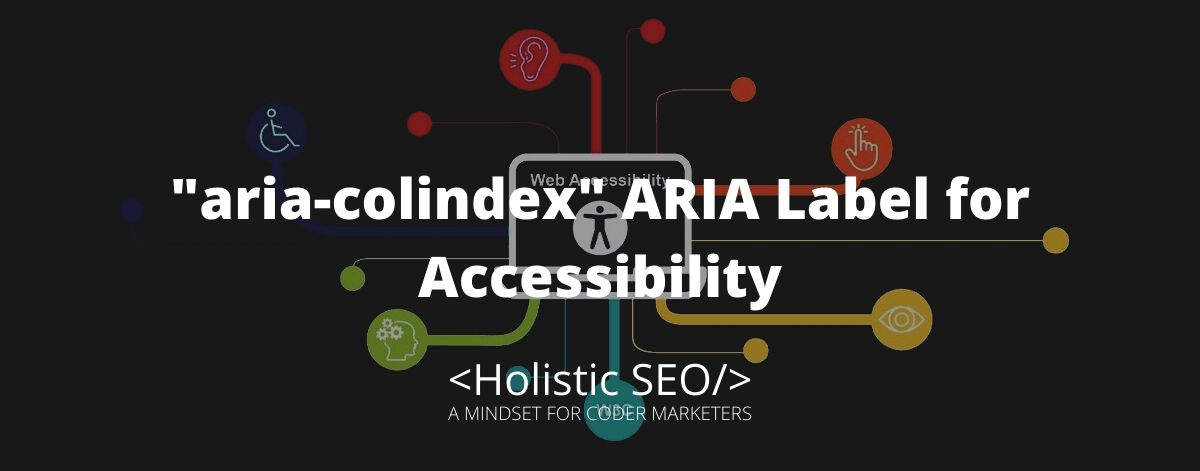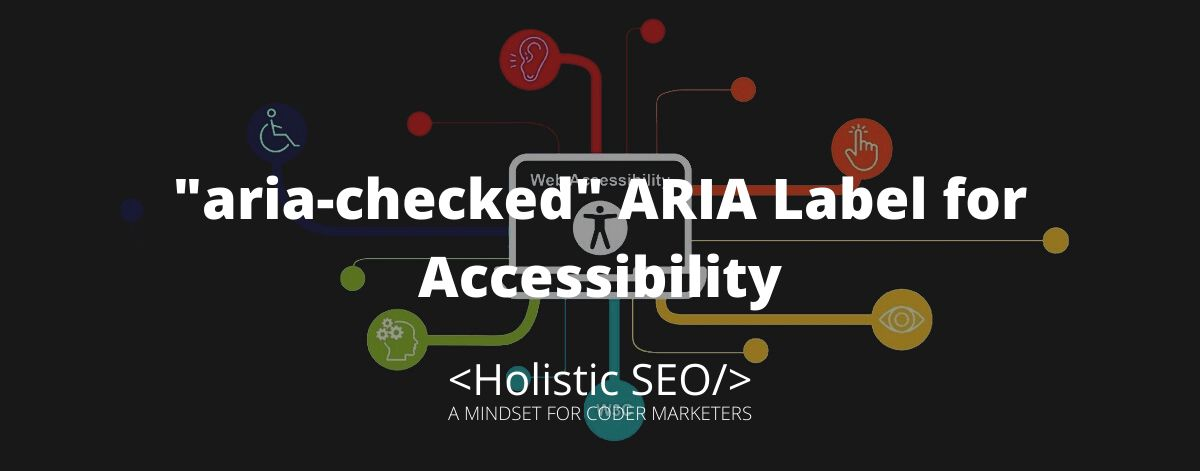# “aria-colcount” ARIA Label for Accessibility

The aria-colcount attribute identifies the total number of columns in a table, grid, or treegrid when not all columns are present in the DOM. The goal of aria-colcount is to show the number of columns the table has. The developer can use the aria-colcount attribute to determine or let the assistive technologies know the number of columns the table has if all columns were present. The value is an integer that serves as the number of columns within the table. The aria-colcount attribute can be used in elements such as <table>, <th>, and <td>. <table> HTML element serves as tabular data, which is information presented in a two-dimensional table made of rows and columns of cells containing data. On the other hand, <th> an HTML element presents a cell as a header of a group of table cells while <td> an HTML element specifies a cell of a table that contains data. The aria-colcount can be used for ARIA Labels like aria-colindex, aria-colindextext, and aria-colspan.

Contents of the Article

## What is the function of the “aria-colcount” ARIA Label?

The main function of the aria-colcount is to let the assistive technologies know the number of columns the table would have if every column is present. If the developer doesn’t know the total number of columns a table will have, but knows they will not all be in the DOM, use the value-1, so aria-colcount”=1”. This value tells the user agent that the actual number of columns present in the DOM may differ from the number of columns in the table. However, if all columns in a table are in the DOM, the aria-colcount attribute isn’t necessary, because browsers immediately calculate the total number of columns.

## What are the uses of the “aria-colcount” ARIA Label?

Listed below are the uses of the “aria-colcount” ARIA Label.

• Table: The table value of the ARIA role attribute indicates that the element comprises the role as having a non-interactive table structure that has data organized in rows and columns, such as the native <table> HTML element.
• Grid: The grid role is for a widget that has one or more rows of cells. The place of each cell is essential and can be adjusted using keyboard input.
• Treegrid: The treegrid role determines an element being a grid whose rows can be elaborated and broken down in the same manner as for a tree.

## Example Uses of “aria-colcount” ARIA Label

Listed below are the example uses of the “aria-colcount” ARIA Label.

When using a semantic table header, elements and not every column are usually found in the DOM. The aria-colcount must still be utilized, but the aria-colindex command needs to be specified once per column in the column header. The example below shows a grid that has 6 columns, wherein columns 1,2,5, and 6 are all presented. The overall number of columns in the table is set as aria-colcount=”6”. The columns aren’t immediate. Each cell found in this case column header and gridcell elements has the aria-colindex command set.

``````<table aria-colcount="6">
<tr>
<th aria-colindex="1" scope="col">First name</th>
<th aria-colindex="2" scope="col">Last name</th>
<th aria-colindex="5" scope="col">City</th>
<th aria-colindex="6" scope="col">Zip</th>
</tr>
<tbody>
<tr>
<td>Martha</td>
<td>Stuart</td>
<td>California</td>
<td>1728</td>
</tr>
...
</tbody>
</table>``````
``````<table aria-colcount=8">
<tr>
<th aria-colindex="3" scope="col">First name</th>
<th aria-colindex="4" scope="col">Last name</th>
<th aria-colindex="5" scope="col">City</th>
<th aria-colindex="7 scope="col">Zip</th>
</tr>
<tbody>
<tr>
<td>Jason</td>
<td>Mary</td>
<td>Jackson</td>
<td>182827</td>
</tr>
...
</tbody>
</table>``````
``````<table aria-colcount="10">
<tr>
<th aria-colindex="1" scope="col">First name</th>
<th aria-colindex="5" scope="col">Last name</th>
<th aria-colindex="7" scope="col">City</th>
<th aria-colindex="10" scope="col">Zip</th>
</tr>
<tbody>
<tr>
<td>Dylan</td>
<td>Jason</td>
<td>1334</td>
</tr>
...
</tbody>
</table>``````
``````<table aria-colcount="6">
<tr>
<th aria-colindex="1" scope="col">First name</th>
<th aria-colindex="2" scope="col">Last name</th>
<th aria-colindex="5" scope="col">City</th>
<th aria-colindex="6" scope="col">Zip</th>
</tr>
<tbody>
<tr>
<td>Allison</td>
<td>John</td>
<td>New York</td>
<td>14127</td>
</tr>
...
</tbody>
</table>``````
``````<table aria-colcount="8">
<tr>
<th aria-colindex="2" scope="col">First name</th>
<th aria-colindex="3" scope="col">Last name</th>
<th aria-colindex="5" scope="col">City</th>
<th aria-colindex="7" scope="col">Zip</th>
</tr>
<tbody>
<tr>
<td>Ronoa</td>
<td>Hiro</td>
<td>Jason</td>
<td>272</td>
</tr>
...
</tbody>
</table>``````

## What are the related ARIA Labels to “aria-colcount”?

Listed below are the related ARIA Labels to “aria-colcount”.

• aria-colindex: The aria-colindex is related to aria-colcount because both attributes specify and determine the total number of columns or index in a table, grid, or treegrid. The aria-colindex indicates an element’s column index or position with regard to the total number of columns.
• aria-colindextext: The aria-coldindextext is related to aria-colcount because they both use the same DOM. The difference with aria-colindextext is that it determines a human-readable text alternative to the numeric aria-colindex. aria-colindextext attribute dictates a human-readable text alternative for the numeric aria-colindex.
• aria-rowindextext: The aria-rowindextext and aria-colcount have similar purposes. They both count columns and rows and define them into a human-readable text alternative. The aria-rowindextext attribute indicates a human-readable text and alternative of aria-rowindex.